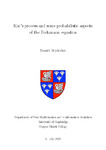## Kac's process and some probabilistic aspects of the Boltzmann equation

##### Authors
Heydecker, Daniel
##### DOIThesis
##### Abstract

We consider a family of stochastic interacting particle systems introduced by Kac as a model for a spatially homogeneous gas undergoing elastic collisions, corresponding to the spatially homogeneous Boltzmann equation. We consider Kac's problem of showing propagation of chaos - that if the velocities of the particles are initially approximately independent, then the same is true at later times - which is equivalent to the convergence of the empirical measures, and which derives the spatially homogeneous Boltzmann equation from the underlying molecular dynamics.

The first two results concern the propagation of chaos for different Kac models. In the first case, we consider the hard spheres kernel, which is appropriate for modelling interactions arising from localised interactions. For this model, we build on previous analyses of the same problem to show that the expected deviation between the Kac process and the Boltzmann equation, measured in expected Wasserstein distance, is of the order N−α. Particular care is paid to the time-dependence of the estimates, using the stability properties of the Boltzmann equation: we will show that the expected deviation at a single fixed time is typically of the order N−α, uniformly in time, while for the largest deviation on a time interval [0,tfin], there is a prefactor (1+tfin)β, where β>0 can be chosen as close to 0 as desired. We also show that similar estimates hold, possibly for smaller α, as soon as the initial data are only assumed to have 2+ϵ moments.

We next consider the case of non-cutoff hard potentials, which arise from modelling a family of long-range interactions. In this case, the collision kernel is doubly-unbounded, both unbounded as the relative velocity increases, and with a non-integrable angular singularity, so that every particle undergoes infinitely many collisions on any nontrivial time-interval. In this context, we introduce a Tanaka-style coupling for a well-chosen distance function on Rd, which allows us to exploit a negative Povzner-type term. This leads to a proof of propagation of chaos and a new uniqueness and stability result for the corresponding Boltzmann equation, assuming only that the initial measure is a probability measure with finitely many moments, whereas previously established results have required either some additional regularity or an exponential moment. In the case of the corresponding hard-potential Landau equation, the same argument can be further refined to show uniqueness and stability for probability measures with 2+ϵ moments. We also show that energy-conserving solutions to the Landau equation exist, assuming only that the initial data has finite energy, and we use the new uniqueness result to show that all solutions to the Landau equation immediately admit analytic densities, aside from the degenerate cases where the initial data is a point mass. By contrast, previous results have only shown that such regular solutions exist.

We further study the dynamical large deviations of the N-particle system, either for the case of a cutoff Maxwell molecules kernel, or a caricature of the hard spheres kernel, for a range of initial conditions including equilibrium. We seek large deviation estimates jointly with an auxiliary flux measure which records the collision history. We prove a large deviation upper bound, and a lower bound restricted to classes of sufficiently regular paths, with a rate function analagous to those found elsewhere in the literature for similar problems. However, we show by exhibiting a family of counterexamples that this rate function does not capture all possible large deviation behaviours; although the particle system almost surely conserves energy, possible large deviation behaviour includes energy non-conserving solutions to the Boltzmann equation, as found by Lu and Wennberg, but these occur strictly more rarely than predicted by the rate function.

The final section concerns Smoluchowski and Flory coagulation equations with a particular bilinear form, which arise when studying the interaction structure of the Kac process by forming clusters of particles joined by chains of collisions, corresponding to the cumulant expansion. Exploiting the bilinear structure and a coupling to random graphs, we are able to give a detailed analysis of the coagulation particle system and limiting Flory equation, including showing the emergence of a unique macroscopic cluster at a finite time tg∈(0,∞), which we characterise exactly in the case of the particle system.

2021-07-06